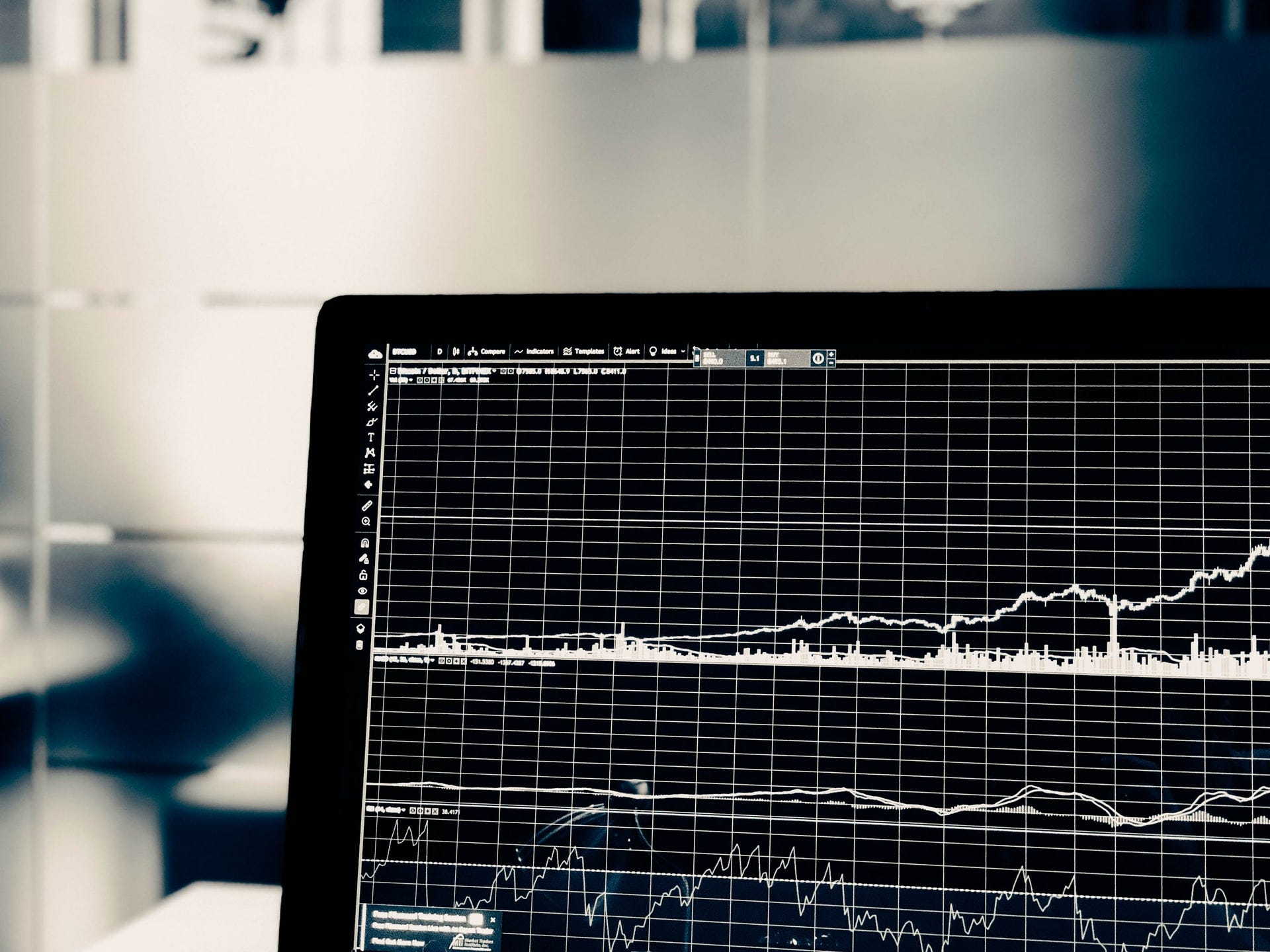# The difference between the mean and the median.

Original article was published by Alvaro Durán Tovar on Deep Learning on Medium# The difference between the mean and the median.

Both aims to represent the “center” of some data. A proper way to define them might be that they define the location of a distribution, defining location as the middle point of it, or the position at which most of the data is located.

# What’s the mean?

First thing to say is that there are multiple kinds of means, the most common is called the arithmetic mean, but there are others called geometric mean, weighted mean, harmonic mean or even the root mean square… all trying to summarize some sense os “middle” of the data considering the whole data, in different ways.

And that’s the key difference with the median. All previous kinds of means mentioned above are calculated considering the whole data you have available:

• Sum everything and divide by it’s cardinality…
• Multiply everything…
• square everything, sum it and then apply root square…

Doing some calculation over each data point and reducing it into 1 number. For the arithmetic mean, the “standard” we typically use, the formula is as:

# What’s the median?

The median give us another way to get the center of the data, and it’s literally the center. The median is calculated by ordering all numbers and finding the value at the middle. Some examples:

• The median of [0,1,2,3,4] is 2.
• The median of [100, 1000, 10000, 100000, 1000000] is 10000.
• The median of [-100, -5, -4.3, 1.2, 1.8] is -4.3.

That was simple because we have an odd number of numbers (take the one in the middle), but what if we have an even number of numbers? Then we take the two that falls on the center and calculate the arithmetic mean:

• The median of [0, 1, 2, 3] is 1.5.
• The median of [100, 1000, 10000, 100000] is 5500.
• The median of [-100, -5, -4.3, 1.2] is -4.65.

# Normal distribution

A normal distribution is parameterized by the mean and the standard deviation, sometimes called location and scale. For this distribution the mean and the median falls in the same place, why? Because it’s symmetrical:

# Other distributions

For other distributions we can see the mean is shifted towards the tail.

And here you can start to have an idea of why this is important. If we have extreme values the mean will move, while the median not. Classic example:

There are 4 guys on a pub drinking a pint of beer. To keep it simple say all of them have a monthly income of 1,000€. The mean is 1,000€ and the median is 1,000€.

Then Bill Gates enters the pub, say he have a monthly income of 1,000,000€ (who knows!).

Now the mean is 200,800€, while the median is still 1,000€.

In cases like this where you have big outliers you should be using the median most likely to have a sense of where is the “middle”.

# When to use each

Having outliers isn’t bad per se and something to avoid at all cost. For example on my job we have done a small change recently that might increase delivery times (it’s a logistic company) in some circumstances by a bit, should I be monitoring the median or the mean? Actually you want to monitor both:

• If the mean goes up means we have extreme values more often or extreme values have gone more extreme if possible.
• If the median goes up means the whole data pattern has changed, everything seems to be affected.

Monitoring the mean is useful to have a sense of how is the data distributed.

Monitoring the median is useful to have a sense of where is the exact midpoint of your data, if it changes something is changing your whole dataset rather than just affecting a subset of it. At the same time, if you don’t want to consider outliers typically the median is the better option.